# SMT-VSL (split from GR and cosmology (split from …A Shrinking matter theory that might actually work.))

## Recommended Posts

If matter shrinks, there must be a reason for that. Some constant would have to vary for this to happen. The “Shrinking Matter Theory with Variable Speed of Light” (SMT-VSL) presents all necessary behavior for that.

This is an alternative theory of the evolution of the universe, which considers the possibility of the evolution of matter over time, which allows the variation of parameters that we consider constant, but that can vary so slowly over time, which is difficult in our lifetime we notice any change. The two main constants that govern the behavior of the universe are the speed of light and Planck's constant. In this Theory we are considering the possibility of the variation of the speed of light, because we know that it is very sensitive to variations in medium, which can be the key to solving the problems found in the theory of the expansion of the universe, thus explaining observed redshift emissions from deep space objects, without the need for its expansion.

The SMT-VSL and the expanding universe theory are equivalent. If we make our world as the reference frame, the universe should expand. If we make the universe as the reference frame, matter should shrink. Laws of physics work to both theories.

The main difference of the expanding universe and the SMT-VSL is what causes the longer wavelength emissions observed of the deep space objects.

The Doppler shift (redshift) is well known in the expanding universe theory.

In the SMT-VSL, the universe is the reference frame, so there is not expansion to cause redshift (except in the systemic local movements like rotation, orbits, binary systems, turbulence, ejection, gravitational effect and gravitational falling), so, the longer wavelengths observed are actually longer emission lines due the bigger size of the atoms in the past.

If we assume the speed of the light varies along the time, and the Planck’s constant keeps the same value, light speed “c” decreases by the factor of  (1+Z)^-(1/3) along the past time, i.e.:

The light speed c(f) in the past frame is c(0) in our local frame, multiplied by the factor (1+Z)^(-1/3).

Then, the notational formula is:    c(f)  = c(o) (1+Z)^(-1/3).

(f) is the reference frame in the past.

(0) is our local frame at present.

Z is the redshift.

The factor (1+Z)^(-1/3)  is not a magic number. It is the factor that enables compatible results of the emission lines and others definitions in the Bohr model.

The hypothesis A is the best in this new theory and it gave us these equations:

c(f) = c(0) (1+Z)^(-1/3)  or c(f )= c(0) [(t+ K(A))/ K(A)] ^(-1/2)

t=K(A) [(1+Z)^(2/3)-1]       Gyr

D= 2K(A) [(1+Z)^(1/3)-1]       Gly

r(f) = r(0)(1+Z)^(2/3) or r(f) = r(0)(t+K(A))/K(A)

c(f): Light speed in a past frame

c(0): Light speed at local frame

t: time (Gyr)

K(A): Contant = 20.657 582 148 024

D: distance (Gly)

r(f): Bohr radius or size of objects in the past (m).

r(0): Bohr radius or size of objects at present (m).

The shrinking speed in one meter is 4.84 nm/C (nanometers per century).

Light speed, in this theory should grow 7.25 mm/s per year.

##### Share on other sites

!

Moderator Note

6 hours ago, Romao Mota said:

If we assume the speed of the light varies along the time, and the Planck’s constant keeps the same value

What happens to the fine structure constant? How quickly does that vary?

If c varies, what is the variation of the permittivity and permeability of free space?

c=1/√(ε0μ0)

##### Share on other sites

6 hours ago, Romao Mota said:

If matter shrinks, there must be a reason for that. Some constant would have to vary for this to happen. The “Shrinking Matter Theory with Variable Speed of Light” (SMT-VSL) presents all necessary behavior for that.

The “speed of light” is a constant by definition and therefore can’t change. Our standard units of length and time and the ratio c are all mutually defined which makes it impossible to measure the speed of light as anything other than what it is defined to be. But you have the right idea that if matter shrinks, the nature of spacetime must change.

The value of c is a constant ratio of distance to time and not necessarily a speed. C can remain a constant if both time and distance remain as the same ratio.

The standard length of a meter is defined as the distance light travels in approximately 1/3x10^8 m/s and a light year is defined as the distance light travels in a year. Consider the impossibility of trying to measure the speed of light in meters or over the distance of a light year.

6 hours ago, Romao Mota said:

The SMT-VSL and the expanding universe theory are equivalent. If we make our world as the reference frame, the universe should expand. If we make the universe as the reference frame, matter should shrink. Laws of physics work to both theories.

This is simple logic that needs no explanation but it is impossible to explain it to anyone who doesn’t immediately find it intuitive. The more one tries to explain it, the more incomprehensible it becomes. If you haven’t discovered this yet... you will.

6 hours ago, Romao Mota said:

The Doppler shift (redshift) is well known in the expanding universe theory.

The Doppler shift is no longer considered the cause of distant redshifts. The current explanation is that space itself is expanding and light waves are being stretched out by the expansion of space which is a much different explanation but conventional thinking and calculations remain rooted in the Doppler shift paradigm.

6 hours ago, Romao Mota said:

Light speed, in this theory should grow 7.25 mm/s per year.

Light speed can vary from one reference frame to another, but within all reference frames, the value of c as a ratio of distance to time should remain the same.

##### Share on other sites

2 hours ago, bangstrom said:

The “speed of light” is a constant by definition and therefore can’t change.

That's not an actual rebuttal.

You disprove a conjecture by showing that it's not consistent with what we observe.

##### Share on other sites

4 hours ago, swansont said:
!

Moderator Note

What happens to the fine structure constant? How quickly does that vary?

If c varies, what is the variation of the permittivity and permeability of free space?

c=1/√(ε0μ0)

As I said in the first post, "This is an alternative theory of the evolution of the universe, which considers the possibility of the evolution of matter over time, which allows the variation of parameters that we consider constant, but that can vary so slowly over time, which is difficult in our lifetime we notice any change."

The fine-structure constant “α” is a dimensionless value, but it reflects the relationship between the electromagnetic coupling constant ‘e” and ”Ԑₒ”, “h”, and “c”.

e = (2 α Ԑₒ h c)1/2   or   e² = 2 α Ԑₒ h c

As c is variable, result Ԑₒ is also variable, then α should vary at the same rate of c.

Rewriting the expression we have: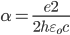But,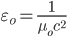And,

μ0 = 4 π (10) -7 = constant

So,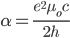Or,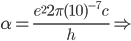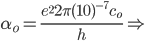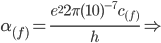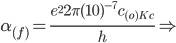α(f)α(o) (Kc)

Or,

α(f)  = α(o) (1+Z)-1/3

α(o)  =  0.007 297 352 5698(24)

“However, if multiple coupling constants are allowed to vary simultaneously, not just α, then in fact almost all combinations of values support a form of stellar fusion."

“Specifically, the values of α, G, and/or c can change by more than two orders of magnitude in any direction (and by larger factors in some directions) and still allow for stars to function."

References:

Michigan Center for Theoretical Physics, Department of Physics, University of Michigan, Ann Arbor, MI 48109          arXiv:0807.3697v1 [astro-ph] 23 Jul 2008

##### Share on other sites

36 minutes ago, Romao Mota said:

As c is variable, result Ԑₒ is also variable, then α should vary at the same rate of c.

It's not obvious that it should vary as the same rate at c. (I assume you mean fractional rate). Since α depends on both c and Ԑₒit should not vary at the same rate as c. IOW, it's not as simple as taking 7.25 mm/s and dividing it by c, since that would imply that Ԑₒis not varying. If it's not, you need to show why all the variation is in the magnetic permeability.

45 minutes ago, Romao Mota said:

μ0 = 4 π (10) -7 = constant

Is it a constant?

##### Share on other sites

2 hours ago, swansont said:

That's not an actual rebuttal.

You disprove a conjecture by showing that it's not consistent with what we observe.

4 hours ago, bangstrom said:

The “speed of light” is a constant by definition and therefore can’t change. Our standard units of length and time and the ratio c are all mutually defined which makes it impossible to measure the speed of light as anything other than what it is defined to be. But you have the right idea that if matter shrinks, the nature of spacetime must change.

The value of c is a constant ratio of distance to time and not necessarily a speed. C can remain a constant if both time and distance remain as the same ratio.

The standard length of a meter is defined as the distance light travels in approximately 1/3x10^8 m/s and a light year is defined as the distance light travels in a year. Consider the impossibility of trying to measure the speed of light in meters or over the distance of a light year.

This is simple logic that needs no explanation but it is impossible to explain it to anyone who doesn’t immediately find it intuitive. The more one tries to explain it, the more incomprehensible it becomes. If you haven’t discovered this yet... you will.

The Doppler shift is no longer considered the cause of distant redshifts. The current explanation is that space itself is expanding and light waves are being stretched out by the expansion of space which is a much different explanation but conventional thinking and calculations remain rooted in the Doppler shift paradigm.

Light speed can vary from one reference frame to another, but within all reference frames, the value of c as a ratio of distance to time should remain the same.

4 hours ago, bangstrom said:

The “speed of light” is a constant by definition and therefore can’t change. Our standard units of length and time and the ratio c are all mutually defined which makes it impossible to measure the speed of light as anything other than what it is defined to be. But you have the right idea that if matter shrinks, the nature of spacetime must change.

The value of c is a constant ratio of distance to time and not necessarily a speed. C can remain a constant if both time and distance remain as the same ratio.

The standard length of a meter is defined as the distance light travels in approximately 1/3x10^8 m/s and a light year is defined as the distance light travels in a year. Consider the impossibility of trying to measure the speed of light in meters or over the distance of a light year.

This is simple logic that needs no explanation but it is impossible to explain it to anyone who doesn’t immediately find it intuitive. The more one tries to explain it, the more incomprehensible it becomes. If you haven’t discovered this yet... you will.

The Doppler shift is no longer considered the cause of distant redshifts. The current explanation is that space itself is expanding and light waves are being stretched out by the expansion of space which is a much different explanation but conventional thinking and calculations remain rooted in the Doppler shift paradigm.

Light speed can vary from one reference frame to another, but within all reference frames, the value of c as a ratio of distance to time should remain the same.

As I said in the first post, "This is an alternative theory of the evolution of the universe, which considers the possibility of the evolution of matter over time, which allows the variation of parameters that we consider constant, but that can vary so slowly over time, which is difficult in our lifetime we notice any change."

The definition of the meter length is an anthropological question. The search for a standard measure has always been a problem in human history. The meter has already gone through several definitions to fix its length. I won't talk about it so as not to be prolix.

One mistake does not justify another. In this theory, the only thing constant in the Universe is the sizes of the electromagnetic waves emitted. In this context, the only standard measure is the peak of the CMB.

##### Share on other sites

6 hours ago, swansont said:
8 hours ago, bangstrom said:

The “speed of light” is a constant by definition and therefore can’t change.

That's not an actual rebuttal.

You disprove a conjecture by showing that it's not consistent with what we observe.

My statement was a comment and not an “actual rebuttal” because the OP makes sense. The “speed of light” can vary from one reference frame to another but the observed value for c should be the same in all individual reference frames.

8 hours ago, swansont said:

c=1/√(ε0μ0)

If c is a constant, then (ε0μ0) is also a constant. Separately, e0 and μ0 may be variables but their multiples and ratios should be a constant, just as when c= d/t. Distance and time may be variables but their observed ratio is a constant.

This implies that c can serve as a conversion factor for converting between units of distance and units of time. The value of c is essentially the length of a standard meter expressed in seconds.

2 hours ago, Romao Mota said:

One mistake does not justify another. In this theory, the only thing constant in the Universe is the sizes of the electromagnetic waves emitted. In this context, the only standard measure is the peak of the CMB.

If the sizes of the EM waves have remained constant, then why do present day emissions appear shorter than primal emissions from the CMB? Your model doesn’t have an expanding space to explain why light waves from distant sources have become “stretched out.” Unless, by constant you mean proportional to the shrinking atomic scale.

##### Share on other sites

5 hours ago, swansont said:

It's not obvious that it should vary as the same rate at c. (I assume you mean fractional rate). Since α depends on both c and Ԑₒit should not vary at the same rate as c. IOW, it's not as simple as taking 7.25 mm/s and dividing it by c, since that would imply that Ԑₒis not varying. If it's not, you need to show why all the variation is in the magnetic permeability.

Is it a constant?

The rate of change of α is described in the answer edited later. α(f)  = α(o) (1+Z)-1/3.  This rate is the same of the variation of “c”, then, in function of time, this rate also would be: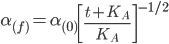If there are any errors, please let me know and I will correct them.

The permeability of free space (a vacuum ) is a physical constant equal to approximately 1.257 x 10 -6 henry per meter . It is symbolized µ o . Permeability in general is symbolized µ, and is a constant of proportionality that exists between magnetic flux density and magnetic field strength in a given medium.

µ o  is a dimensionless constant.

μ0 = 4 π (10) -7

Vacuum permittivity, commonly denoted ε0 (pronounced as "epsilon nought" or "epsilon zero") is the value of the absolute dielectric permittivity of classical vacuum. Alternatively may be referred to as the permittivity of free space, the electric constant, or the distributed capacitance of the vacuum. It is an ideal (baseline) physical constant. Its CODATA value is: 

ε0 = 8.8541878128(13)×10−12 Fm−1So, ε(f) in a past frame would be: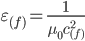But, c(f)=c(0)(1+Z) -1/3 =>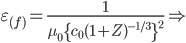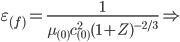ε(f)= ε(0)(1+Z)2/3

This rate is the same of the variation of the size of bodies and atoms “r”, then, in function of time, ε(f) also would be:

ε(f)= ε(0) (t+K(A)) / K(A)

The change in the vacuum permittivity is what allows “c” to vary along the time. This variation is actually the evolution of the universe. The redshift of the emissions in the past is the observational data of that.

References:

##### Share on other sites

7 hours ago, bangstrom said:

If the sizes of the EM waves have remained constant, then why do present day emissions appear shorter than primal emissions from the CMB? Your model doesn’t have an expanding space to explain why light waves from distant sources have become “stretched out.” Unless, by constant you mean proportional to the shrinking atomic scale.

In the first post, I said “In the SMT-VSL, the universe is the reference frame, so there is not expansion to cause redshift (except in the systemic local movements like rotation, orbits, binary systems, turbulence, ejection, gravitational effect and gravitational falling), so, the longer wavelengths observed are actually longer emission lines due the bigger size of the atoms in the past.” They are not "stretched out".

CMB is not a consensus in the scientific community. It is “almost” impossible for anyone to know its origin, which material emitted this energy. I don't know of any research indicating CMB emission in wavelenght "shorter than primal emissions" in our local frame.

##### Share on other sites

4 hours ago, Romao Mota said:

In the first post, I said “In the SMT-VSL, the universe is the reference frame, so there is not expansion to cause redshift

It is clear that light waves are not “stretched out” in your model but I was asking if light wave emissions are growing shorter with time? That is, progressively shorter starting with the CMB and up to the present time.

##### Share on other sites

15 hours ago, bangstrom said:

My statement was a comment and not an “actual rebuttal” because the OP makes sense. The “speed of light” can vary from one reference frame to another but the observed value for c should be the same in all individual reference frames.

And they are advancing a conjecture that it is not, so the statement has little meaning.

9 hours ago, Romao Mota said:

The rate of change of α is described in the answer edited later. α(f)  = α(o) (1+Z)-1/3.  This rate is the same of the variation of “c”, then, in function of time, this rate also would be:If there are any errors, please let me know and I will correct them.

A. I'm asking for a number. You gave a number for c

B. How can it be the same as the rate of change of c?  Ԑₒ  and c are both in the equation for α. If α varies as c does, then Ԑₒ can't be changing. But c depends on Ԑₒand you've said that μ0 is a constant. This is mathematically inconsistent.

C. Presenting the same information over and over again isn't going to be fruitful. Repeating is not clarification.

9 hours ago, Romao Mota said:

The permeability of free space (a vacuum ) is a physical constant equal to approximately 1.257 x 10 -6 henry per meter . It is symbolized µ o . Permeability in general is symbolized µ, and is a constant of proportionality that exists between magnetic flux density and magnetic field strength in a given medium.

µ o  is a dimensionless constant.

You just said that the units of μare H/m, which is correct. Thus it is not a dimensionless constant.

If c varies as 7.25 mm/s per year, then the fractional change is 2.4 x 10^-11 per year. If α is changing at this rate, we have a problem,

"In 2008, Rosenband et al. used the frequency ratio of Al+ and Hg+ in single-ion optical atomic clocks to place a very stringent constraint on the present-time temporal variation of α, namely α̇/α = (−1.6±2.3)×10−17 per year. "

##### Share on other sites

On 5/8/2022 at 11:11 PM, Romao Mota said:

The SMT-VSL and the expanding universe theory are equivalent.

The value of having an equivalent but alternative model to the Standard BB expansion theory is that we can compare one model with the other looking for consensus and also looking for inconsistencies that suggest there may be something wrong with our interpretation of one or both models. I think your model of shrinking matter model suggests some serious problems with the expansion theory but it works both ways.

I hope I understand how you find speed of light to be varying in your model but if you apply the same thinking the SMBB you should find that the speed of light is also varies from reference frame to reference frame in the Standard Model. If space expands while time remains the same, the speed of light must necessarily be slowing.

My understanding of this is that your model is no more a model of changes in the speed of light than is the SMBB, since in both models, the speed of light should be measured the same in each and every individual reference frame.

So how is your model any different from the SMBB? A little known mathematician named Russel Ryerson had a model of contracting matter essentially the same as yours and he said one model is the conceptual and mathematical inverse of the other. This is why they are equivalent and he called his model “Inverse Expansion.”

In the SMBB, space is expanding while time remains the same. In your model, matter is contracting, while space remains the same. I explained earlier in my comment of “little meaning” that the measured value of c remains the same in every reference frame and this should apply to your model just as it does to the SMBB. So your model is one of shrinking matter rather than a changing speed of light.

As particles in the material world grow smaller, they spin faster, and our perception of time quickens.

In the SMBB model, space expands while time remains the same and the speed of light slows from one reference frame to another. In your model, space remains the same while matter contracts and the speed of light quickens from one reference frame to another. One is the simple inverse of the other but we can't go back in time and measure light speed in these older reference frames so the variations are safely ignored.

Our observation of c remains the same in both models so neither one can be considered a model of a variable speed of light. The real difference is that the SMBB is a model of expanding space and yours is a model of quickening time.

##### Share on other sites

On 5/9/2022 at 12:11 AM, Romao Mota said:

If we make our world as the reference frame, the universe should expand. If we make the universe as the reference frame, matter should shrink. Laws of physics work to both theories.

That's not true.
GR does not allow for a universal reference frame, so it would not be consistant with the latter case.

##### Share on other sites

3 hours ago, MigL said:

That's not true.
GR does not allow for a universal reference frame, so it would not be consistant with the latter case.

Very true about GR, but relative to ‘What’ is the universe expanding? Or is it expanding at all?

I had a goldfish that grew too large for its bowel, but from the perspective of a goldfish, the bowel must have been growing too small for the fish.

When a woman puts on a pair of old pair of pants and the pants are too tight, she thinks she must be getting fat. When a man puts on a pair of old pair of pants and the pants are too tight, he thinks the pants must have shrunk.

With the universe as a whole, we don’t have a god’s eye view of events where we can view the universe from the great beyond and watch it expand or contract or remain the same so we have no external scale to tell us if the universe is truly expanding or if all the material within is growing smaller.

We are like goldfish in a bowel where our only perspective is that of what the universe looks like from the inside and our choice of absolutes is arbitrary because the laws of physics and math are blind to perspective and they do work both ways.

##### Share on other sites

7 hours ago, bangstrom said:

We are like goldfish in a bowel where our only perspective is that of what the universe looks like from the inside and our choice of absolutes is arbitrary

Unlike the goldfish' s situation, that is the only perspective there is.

##### Share on other sites

16 hours ago, bangstrom said:

can't go back in time and measure light speed in these older reference frames so the variations are safely ignored.

But we actually can “go back in time” for measurements because of the finite speed of light. Light from distant sources are a record of interactions that took place millions or billions of years ago.

##### Share on other sites

On 5/10/2022 at 7:20 AM, swansont said:

And they are advancing a conjecture that it is not, so the statement has little meaning.

A. I'm asking for a number. You gave a number for c

B. How can it be the same as the rate of change of c?  Ԑₒ  and c are both in the equation for α. If α varies as c does, then Ԑₒ can't be changing. But c depends on Ԑₒand you've said that μ0 is a constant. This is mathematically inconsistent.

C. Presenting the same information over and over again isn't going to be fruitful. Repeating is not clarification.

You just said that the units of μare H/m, which is correct. Thus it is not a dimensionless constant.

If c varies as 7.25 mm/s per year, then the fractional change is 2.4 x 10^-11 per year. If α is changing at this rate, we have a problem,

"In 2008, Rosenband et al. used the frequency ratio of Al+ and Hg+ in single-ion optical atomic clocks to place a very stringent constraint on the present-time temporal variation of α, namely α̇/α = (−1.6±2.3)×10−17 per year. "

You were right, μ0 really is not dimensionless, μr is.

In the research by Rosenband et al 2008they used atomic clocks to measure atomic properties of aluminum and mercury.

Aluminum, Mercury and Cesium133  are made up of atoms that are made of the same stuff, protons, neutrons and electrons. Considering that both the three are increasing the frequency of their emissions at the same rate, the best expected result would be a null value, (zero) variation in α, which in this case is consistent with the result, since the tolerance is greater than the median variation.

The work of Rosenband et al 2008 would makes sense to test the accuracy of the equipment used, because, for a predicted result of null variation, the result of (−1.6±2.3)×10−17 per year is very close to the expected null value.

Atomic clocks are very accurate and important, but to measure variations in atomic behavior, it is necessary to calibrate the clock to an invariant frequency in the universe. In SMT-VSL, we have two frequencies that are immutable, which are the PEAK of the CMBs and the PEAK of the unresolved CXRBs.

Below we have a table with the main formulas used in SMT-VSL, and a partial  image of an excel plan with some “numbers” you asked for.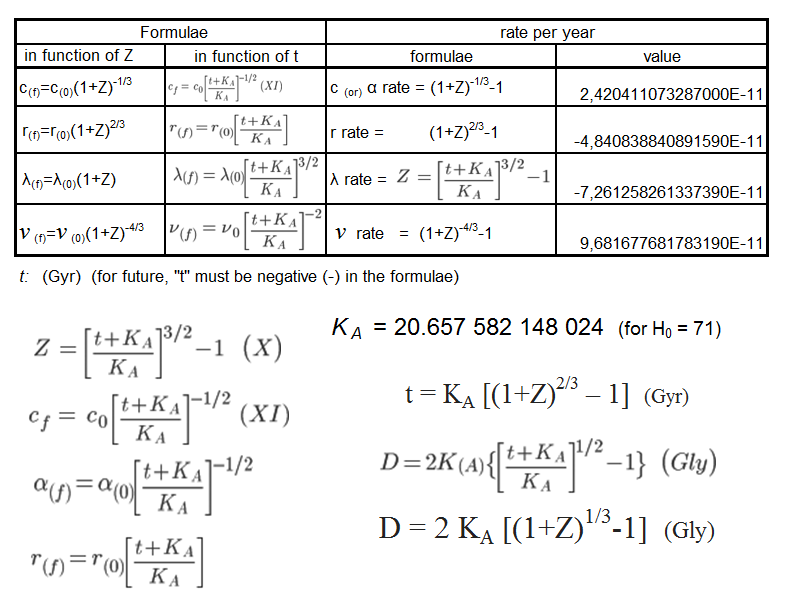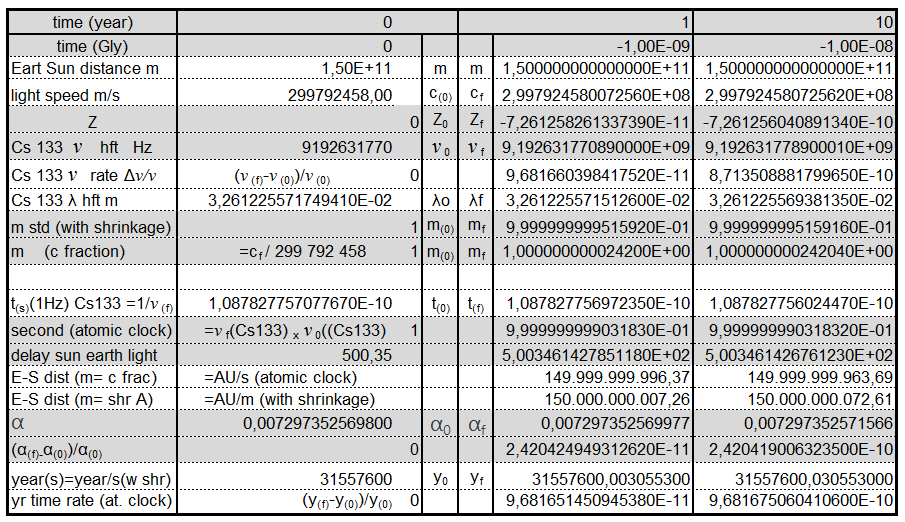##### Share on other sites

13 hours ago, Romao Mota said:

Aluminum, Mercury and Cesium133  are made up of atoms that are made of the same stuff, protons, neutrons and electrons. Considering that both the three are increasing the frequency of their emissions at the same rate, the best expected result would be a null value, (zero) variation in α, which in this case is consistent with the result, since the tolerance is greater than the median variation.

You expect a null result only if α does not change, but you are claiming it does change, though you refuse to cite a value.

Quote

Atomic clocks are very accurate and important, but to measure variations in atomic behavior, it is necessary to calibrate the clock to an invariant frequency in the universe.

No, since you are measuring relative values calibration is not needed. When you take the ratio of the frequencies, any calibration factor divides out - it's not part of the result.

You have not addressed the issue of other "constants" changing. Since c=1/√(ε0μ0) what changes will we see in ε0 and μ0? At least one of them has to change if c changes. Ignoring this problem won't make it go away

##### Share on other sites

11 hours ago, swansont said:

You expect a null result only if α does not change, but you are claiming it does change, though you refuse to cite a value.

No, since you are measuring relative values calibration is not needed. When you take the ratio of the frequencies, any calibration factor divides out - it's not part of the result.

You have not addressed the issue of other "constants" changing. Since c=1/√(ε0μ0) what changes will we see in ε0 and μ0? At least one of them has to change if c changes. Ignoring this problem won't make it go away

To measure changes in the properties of atoms along the time, no one can use a clock that also varies with time, since it is made atoms and also varies over time.

Variation of ”ε” was explained in the answer of Sunday, may/08/22  9:54 PM.

The equation c=1/√(ε0μ0) was rewritten in another form:The end result in that post was:

ε(f)= ε(0)(1+Z)2/3

This rate is the same of the variation of the size of bodies and atoms “r”, then, in function of time, ε(f) also would be:

ε(f)= ε(0) (t+K(A)) / K(A)

Below, I have re-edited the two images posted in the previous post, including epsilon ε .

The main formulas used in SMT-VSL, and a partial image of an excel plan with some numbers: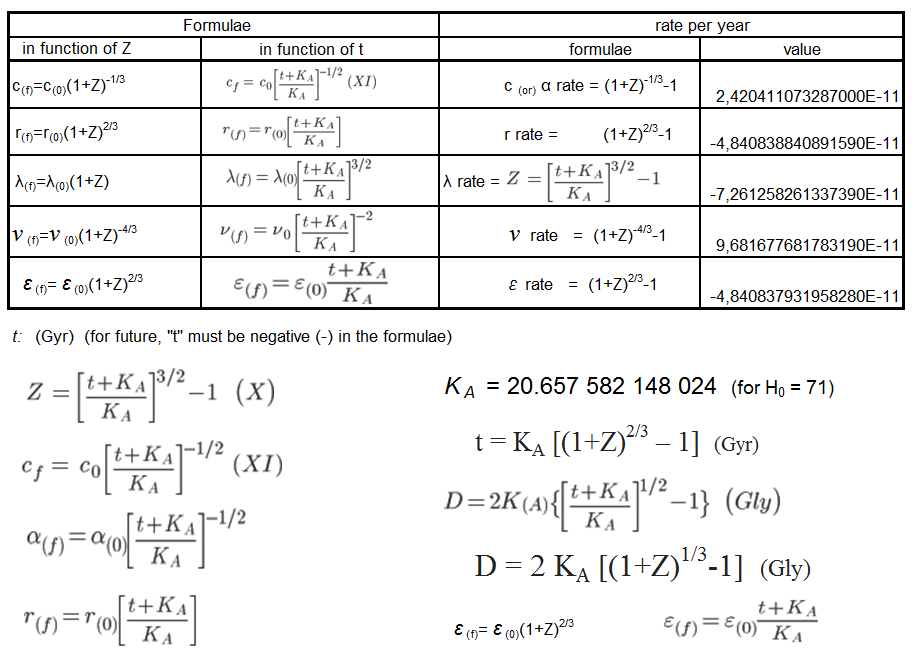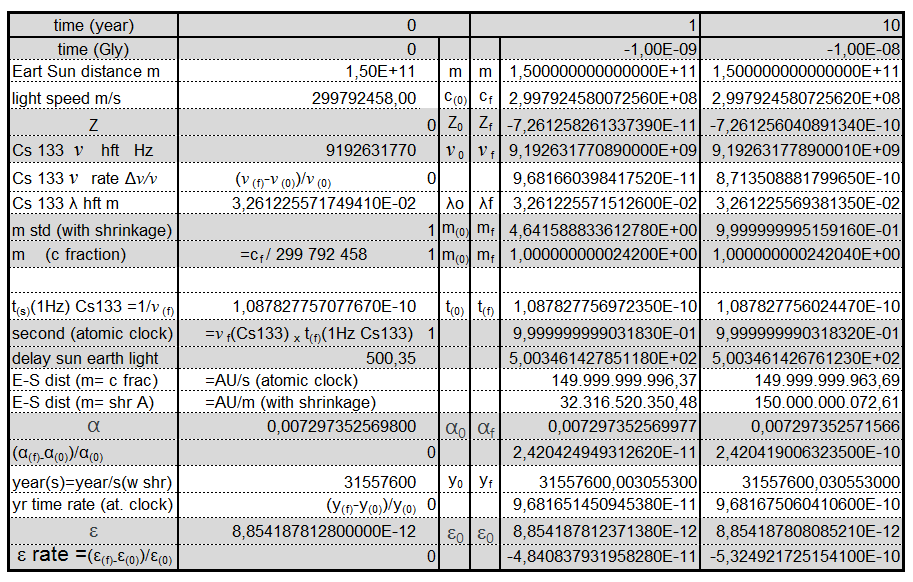##### Share on other sites

17 hours ago, Romao Mota said:

To measure changes in the properties of atoms along the time, no one can use a clock that also varies with time, since it is made atoms and also varies over time.

Variation of ”ε” was explained in the answer of Sunday, may/08/22  9:54 PM.

If c varies and ε varies, then the fine structure variation is not the same as the c variation. That’s simple math.

##### Share on other sites

2 hours ago, swansont said:

If c varies and ε varies, then the fine structure variation is not the same as the c variation. That’s simple math.

Criticisms are welcome and help a lot to correct errors and to increase the deployment of information that only others can see.

This theory follows a line of thought where whenever the constant "c" appears in an equation, it is followed by the constant of variation (1+Z)-1/3. This is simple math. The next step is to rewrite the equation as a function of time. Constants “ε” and "c" have different variables because they appear with different exponentials in that equation, that's all.

I just wanted to clarify information that might not have been noticed.

No one is forced to like or approve of any theory.

Every theory starts from the assumption of the possibility that the event can happen. If someone does not accept the premises, there is nothing to be done.

##### Share on other sites

41 minutes ago, Romao Mota said:

Criticisms are welcome and help a lot to correct errors and to increase the deployment of information that only others can see.

This theory follows a line of thought where whenever the constant "c" appears in an equation, it is followed by the constant of variation (1+Z)-1/3. This is simple math. The next step is to rewrite the equation as a function of time. Constants “ε” and "c" have different variables because they appear with different exponentials in that equation, that's all.

So when they both appear, you have to divide one by the other, and can’t say that the equation varies the same way that c does.

So how does the fine structure constant vary? It’s not (1+Z)-1/3 right? It should vary as (1+Z)+1/3

If the variation depends on Z, then why do you say that c grows by 7.25 mm/s per year? There’s no redshift.

Quote

The factor (1+Z)^(-1/3)  is not a magic number. It is the factor that enables compatible results of the emission lines and others definitions in the Bohr model.

Except that won’t work, because the Bohr energy levels depend on k2 owing to the Coulomb interaction, and k depends on ε0

So you have to account for the change in the energy levels (also the Bohr model isn’t right, but that’s a separate discussion)

## Create an account

Register a new account